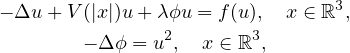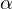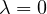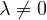Electron. J. Differential Equations, Vol. 2020 (2020), No. 130, pp. 1-17.

### Schrodinger-Poisson systems with singular potential and critical exponent Senli Liu, Haibo Chen, Zhaosheng Feng

Abstract:where V is a singular potential with the parameterand the nonlinearity f satisfies critical growth. By applying a generalized version of Lions-type theorem and the Nehari manifold theory, we establish the existence of the nonnegative ground state solution when. By the perturbation method, we obtain a nontrivial solution to above system when.Senli Liu School of Mathematics and Statistics Central South University Changsha, Hunan 410083, China email: mathliusl@csu.edu.cn Haibo Chen School of Mathematics and Statistics Central South University Changsha, Hunan 410083, China email: math_chb@163.com Zhaosheng Feng School of Mathematical and Statistical Sciences University of Texas Rio Grande Valley Edinburg, TX 78539, USA email: zhaosheng.feng@utrgv.edu